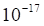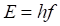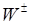Chapter 12, Problem 6C

Chapter
Section
Textbook Problem

In the electroweak theory, symmetry breaking occurs on a length scale of aboutm. Compute the frequency of particles with de Brogue wavelengths (see Section 10.5) equal to this value, and, using this result, determine their energies by the Planck formulaShow that the mass of particles having such energies is on the order of the mass of theparticles (Figure 12.23).

To determine

The frequency of the particle and the energy of it. The mass of the particle is comparable to that of W±.

Explanation

Given:

De-Broglie's wavelength,λ=1017mMass of the particle,m=1.19×1024kgPlank's constant,h=4.13× 10 15eV.s.

Formula used:

Velocity of electromagnetic particle is given by,

c=λf

Where,

λ = De-Broglie’s wavelength

c = velocity of light

f = frequency

Energy of the particle is given by,

E=hf

Where,

E = Energy of the particle.

Calculation:

Velocity of the particle is given by,

c=λff=cλf=c×1017Hz

Energy of the particle is given by,

E=hfE=4

Still sussing out bartleby?

Check out a sample textbook solution.

See a sample solution

The Solution to Your Study Problems

Bartleby provides explanations to thousands of textbook problems written by our experts, many with advanced degrees!

Get Started